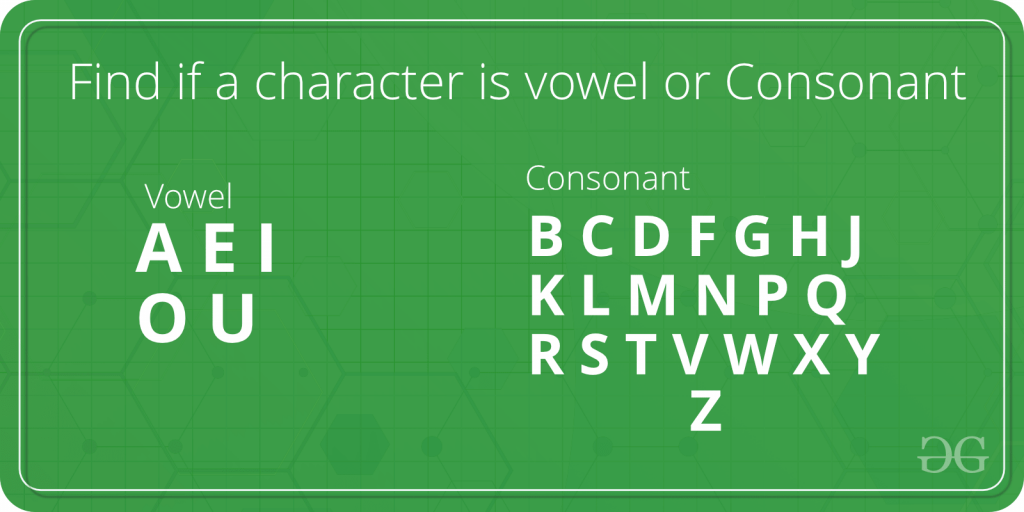# Java Program to Check Whether the Character is Vowel or Consonant

• Difficulty Level : Basic
• Last Updated : 17 Mar, 2021

For any given character, we need to check if it is a vowel or a consonant. As we know, vowels are ‘a’, ‘e’, ‘i’, ‘o’, ‘u’ and all the other characters (i.e. ‘b’, ‘c’, ‘d’, ‘f’ …..) are consonants.Examples:

```Input : char = 'r'
Output : Consonant

Input : char = 'e'
Output : Vowel```

Here, in the below implementation we will check if the stated character corresponds to any of the five vowels. And if it matches, “Vowel” is printed, else “Consonant” is printed.

Example 1:

## Java

 `// java program to check whether input``// character is a vowel or consonant`` ` `import` `java.io.*;`` ` `public` `class` `geek {`` ` `    ``// Function to find whether an input``    ``// character is vowel or not``    ``static` `void` `Vowel_Or_Consonant(``char` `y)``    ``{``        ``if` `(y == ``'a'` `|| y == ``'e'` `|| y == ``'i'` `|| y == ``'o'``            ``|| y == ``'u'``)``            ``System.out.println(``"It is a Vowel."``);``        ``else``            ``System.out.println(``"It is a Consonant."``);``    ``}`` ` `    ``// The Driver code``    ``static` `public` `void` `main(String[] args)``    ``{``        ``Vowel_Or_Consonant(``'b'``);``        ``Vowel_Or_Consonant(``'u'``);``    ``}``}`

Output

```It is a Consonant.
It is a Vowel.```

Example 2:

• Alteration for capital letters.

## Java

 `// java program to check whether input``// character is a vowel or consonant`` ` `import` `java.io.*;`` ` `public` `class` `geek {`` ` `    ``// Function to find whether an input``    ``// character is vowel or not``    ``static` `void` `Vowel_Or_Consonant(``char` `y)``    ``{``        ``if` `(y == ``'a'` `|| y == ``'e'` `|| y == ``'i'` `|| y == ``'o'``            ``|| y == ``'u'` `|| y == ``'A'` `|| y == ``'E'` `|| y == ``'I'``            ``|| y == ``'O'` `|| y == ``'U'``)``            ``System.out.println(``"It is a Vowel."``);``        ``else``            ``System.out.println(``"It is a Consonant."``);``    ``}`` ` `    ``// The Driver code``    ``static` `public` `void` `main(String[] args)``    ``{``        ``Vowel_Or_Consonant(``'W'``);``        ``Vowel_Or_Consonant(``'I'``);``    ``}``}`

Output

```It is a Consonant.
It is a Vowel.```

Example 3:

## Java

 `// java program to check whether input``// character is a vowel or consonant`` ` `import` `java.io.*;`` ` `class` `GFG {``    ``// Function to find whether an input``    ``// character is vowel or not``    ``static` `String isVowel(``char` `ch)``    ``{``        ``// Make the list of vowels``        ``String str = ``"aeiouAEIOU"``;``        ``return` `(str.indexOf(ch) != -``1``) ? ``"Vowel"``                                       ``: ``"Consonant"``;``    ``}`` ` `    ``// Driver Code``    ``public` `static` `void` `main(String[] args)``    ``{``        ``System.out.println(``"It is a "` `+ isVowel(``'a'``));``        ``System.out.println(``"It is a "` `+ isVowel(``'x'``));``    ``}``}`

Output

```It is a Vowel
It is a Consonant
```

My Personal Notes arrow_drop_up